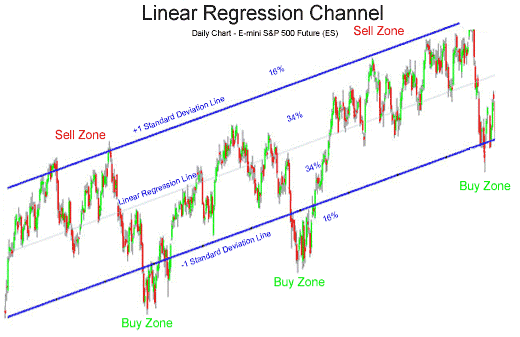top of page
• Peet Serfontein

# Understanding Linear Regression Channels and Standard Deviation in Price Action Analysis

Introduction In the world of technical analysis, traders and investors often use various tools and indicators to gain insights into market trends and potential price movements. One such tool is the Linear Regression Channel, which utilises statistical concepts like standard deviation to help identify the potential range of price action. Understanding how to interpret and use the Linear Regression Channel in conjunction with standard deviation can provide valuable information for traders to make informed decisions.

What is Linear Regression Channel? The Linear Regression Channel is a technical analysis tool that plots a straight line based on the linear regression of the price data over a specific period. This line serves as the central axis of the channel, with two parallel lines drawn above and below it, representing the upper and lower ranges. The channel helps traders visualize the general direction of the trend and possible areas of support and resistance. Calculating the Linear Regression Channel involves fitting a linear regression line to the price data, usually based on the closing prices, over a defined look-back period. The resulting line represents the average or mean of the price data over that period.Understanding Standard Deviation Standard deviation is a measure of the dispersion or variability of the price data around the linear regression line. In the context of a Linear Regression Channel, standard deviation helps determine the width of the channel, which indicates the potential volatility or uncertainty of price movements.

When the standard deviation is high, it means the price data is spread out widely from the regression line, indicating higher volatility. Conversely, a low standard deviation suggests that the price data is closely clustered around the regression line, indicating lower volatility.

Using Scoring to Assess Proximity to the Linear Regression Channel The term "scoring" in this context refers to a numerical value representing the distance of the current price from the linear regression line. This score is typically normalised to a scale between 0 and 10, with 1 indicating the lowest proximity to the regression line and 10 indicating the highest proximity. The relationship between the scoring and the Linear Regression Channel is as follows:

1. Scoring Close to 0: When the scoring is closer to 0, it suggests that the current price is near the lower range of the Linear Regression Channel. This indicates that the market is potentially oversold, and the price may be due for a correction or bounce towards the mean or central axis of the channel.

2. Scoring Close to 10: Conversely, when the scoring is closer to 10, it suggests that the current price is near the upper range of the Linear Regression Channel. This indicates that the market is potentially overbought, and the price may be due for a correction or a bounce back towards the mean.

Interpreting Linear Regression Channel and Standard Deviation Together Combining the Linear Regression Channel and standard deviation provides a more comprehensive picture of the market's behaviour. When the standard deviation is wider, the channel broadens, indicating higher volatility. In contrast, a narrower standard deviation results in a narrower channel, suggesting lower volatility.

Traders can use this information to:

• Identify Potential Reversal Zones: When the price action reaches the upper or lower range of the channel, coupled with high standard deviation, it may indicate an overextended market and a potential reversal in the opposite direction.

• Recognize Trend Strength: If the price remains within a narrow channel and the standard deviation is relatively low, it suggests a stable and well-defined trend, while a wide channel with a high standard deviation indicates a more erratic or uncertain market.

Conclusion The Linear Regression Channel and standard deviation are valuable tools for traders to gain insights into the potential range of price action and market volatility. When combined, they can provide a clearer understanding of trend direction, potential reversal points, and overall market sentiment. By using the scoring system to assess proximity to the channel, traders can make more informed decisions, enhancing their ability to navigate the dynamic world of financial markets. However, as with any technical analysis tool, it is essential to use these indicators in conjunction with other forms of analysis and risk management strategies to make well-rounded and informed trading decisions.

Top 40 share scoring and selected markets

The bar chart displayed below presents the constituents of the Top 40 index. This dynamic bar chart is updated weekly, providing valuable insights.